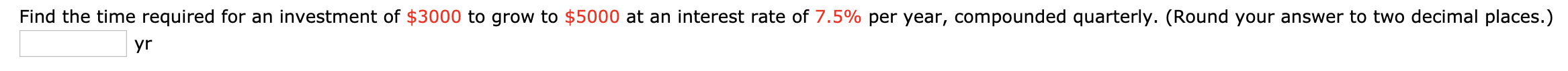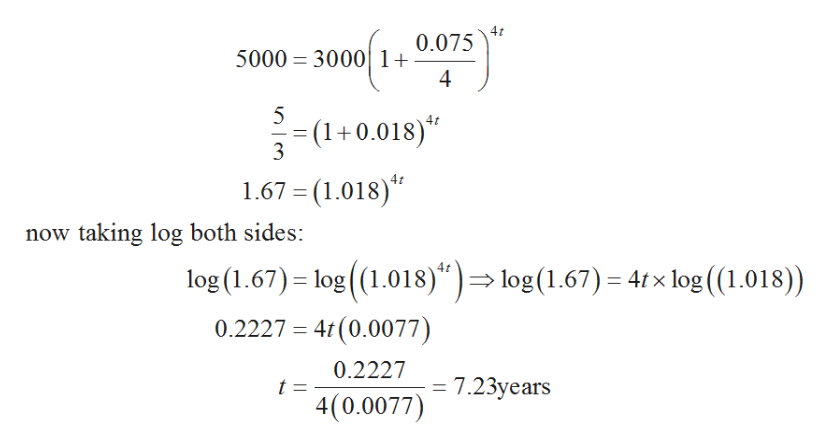# Find the time required for an investment of \$3000 to grow to \$5000 at an interest rate of 7.5% per year, compounded quarterly. (Round your answer to two decimal places.)yr

Question
22 viewshelp_outlineImage TranscriptioncloseFind the time required for an investment of \$3000 to grow to \$5000 at an interest rate of 7.5% per year, compounded quarterly. (Round your answer to two decimal places.) yr fullscreen
check_circle

Step 1

According to the given information:

Principal (P) = \$3000

Amount (A) = \$5000

Rate of interest = 7.5% per annum

It is needed to calculate the time (t) when compounded quarterly.

Step 2

Formula to calculate the amount when compounded quarterly is:

Step 3

Now, plug the values in the gi...help_outlineImage Transcriptionclose4t 0.075 5000 30001 4 5 - (1+0.018) 1.67 (1.018) now taking log both sides: log (1.67) log (1.018)") => log(1.67) = 4tx log (1.018)) 0.2227 4t(0.0077) 0.2227 = 7.23years t = 4(0.0077) fullscreen

### Want to see the full answer?

See Solution

#### Want to see this answer and more?

Solutions are written by subject experts who are available 24/7. Questions are typically answered within 1 hour.*

See Solution
*Response times may vary by subject and question.
Tagged in

### Other